Courses

# Test: Conduction Level - 1

## 20 Questions MCQ Test Engineering Mechanics | Test: Conduction Level - 1

Description
This mock test of Test: Conduction Level - 1 for Chemical Engineering helps you for every Chemical Engineering entrance exam. This contains 20 Multiple Choice Questions for Chemical Engineering Test: Conduction Level - 1 (mcq) to study with solutions a complete question bank. The solved questions answers in this Test: Conduction Level - 1 quiz give you a good mix of easy questions and tough questions. Chemical Engineering students definitely take this Test: Conduction Level - 1 exercise for a better result in the exam. You can find other Test: Conduction Level - 1 extra questions, long questions & short questions for Chemical Engineering on EduRev as well by searching above.
QUESTION: 1

### The general heat conduction equation for 1D heat flow is given as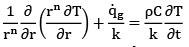For Cartesian coordinates, n is equal to

Solution: For Cartesian coordinates, the general equation for 1D heat conduction is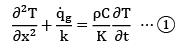We use x in Cartesian coordinates, instead of r. where x represents the length (thickness) of the body. By putting n = 0 in the equation mentioned, we can get the above equation.

QUESTION: 2

### What is the total number of known conditions required to get a particular solution of a 3D unsteady state heat conduction problem

Solution: The 3-D unsteady state heat conduction equation is given as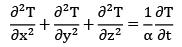For obtaining the particular solution of this equation we need 6 conditions for space coordinates {2 for each coordinate} and 1 initial condition.

QUESTION: 3

### Which of the following options does not follow lumped system analysis during heat or cooling process

Solution: Food items do not follow the lumped system analysis because their thermal conductivity is low, so the temperature inside the food object does not remain uniform. Like when we bite a samosa, it has a warm crust but inner potatoes are hot.

QUESTION: 4

An iron block gets heated up or cools down very fast as compared to water because of __________.

Solution: Iron block cools much faster as compared to water because iron has high thermal diffusivity. Which is the response of a material to applied temperature gradient during unsteady state conditions.

QUESTION: 5

A plane wall is 25 cm thick with an area of , and has a thermal conductivity of 0.5 W/m-K. If a temperature difference of 60°C is imposed across it, what is the heat transfer rate?

Solution: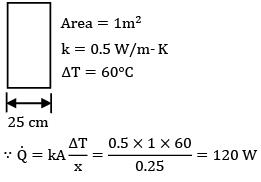QUESTION: 6

For steady state 1D heat conduction through a planar wall with internal heat generation, the temperature profile is

Solution: For the given conditions, the heat conduction equation is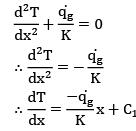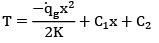QUESTION: 7

What is the expression for resistance against thermal conduction through a hollow sphere of inner radius r1 and outer radius r2, and thermal conductivity k?

Solution: In case of sphere the thermal resistance is given by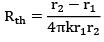Where r1 and r2 are inner and outer radii respectively and k is the thermal conductivity of material

QUESTION: 8

Consider radial steady state heat conduction through a hollow spherical conductor carrying a heat source inside the sphere. The temperature distribution in the shell T = T (r ) is __________

T is the temperature at a distance r from the center.

Solution: In the question, the sphere is carrying a heat source.

General heat conduction equation in spherical coordinates is given as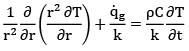Based on conditions of the question, the equation is reduced to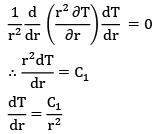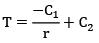Thus T and r follow a hyperbolic relationship.

QUESTION: 9

An electric cable of aluminium conductor (k = 240 W/m-K) is to be insulated with rubber (k = 0.15 W/m-K). The cable is exposed to air (h = 6 W/m2-K). The critical radius of insulation will be

Solution: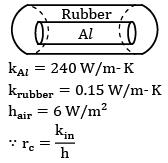Where kin is the thermal conductivity of insulation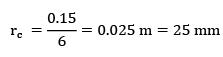QUESTION: 10

Which of the following statements is true regarding fins (extended surfaces for enhanced heat dissipation from a surface)?

Solution: Effectiveness of an infinitely long fin is given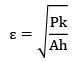Thus fins are more effective if they are made of high conductivity material and thin in cross-section. Also they are more effective in an environment of lower h i.e gases. By placing them closely it becomes possible to incorporate more fins on a single surface enhancing heat dissipation. Thus all three statements are correct.

QUESTION: 11

The figure below shows a cross section of an insulated heating pipe which is made from steel (k = 45 W/m-K) with an inner radius of 150 mm and outer radius of 155 mm. The pipe is coated with 100 mm thickness of insulation having thermal conductivity of k = 0.006 W/m-K.Air at a temperature Ti = 60°C flows through the pipe. The value of corresponding convective coefficient is hi = 35 W/m2-K. The outside surface of the pipe is exposed to air which is 15°C at and convective heat transfer coefficient on this surface has a value of ho = 10 W/m2-K. Find the critical radius of insulation.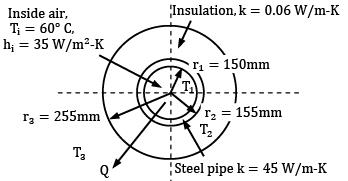Surrounding air T0 = 15°C,ho = 10W/m2-K

Solution: For cylindrical bodies, rc =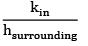Given that kin = 0.06 W/m-K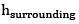= 10 W/m2-K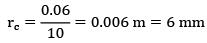QUESTION: 12

The fin efficiency is defined as the ratio of the actual heat transfer from the fin to

Solution:QUESTION: 13

A hot surface at 80°C exposed to air at 20°C is to be cooled by attaching 10-cm-long and 1-cm-diameter cylinder fins. The combined heat transfer coefficient is 30 W/m2 . K, and heat transfer from the fin tip is negligible. If the fin efficiency is 0.75, the rate of heat loss from 100 fins is

Solution: Fin efficiency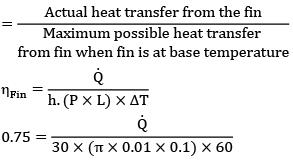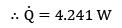Total heat transfer from 100 firms is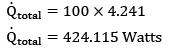QUESTION: 14

The effectiveness (∊) and efficiency (?) of a fin (extended surfaces) can be related as

Solution: Consider an infinitely long fin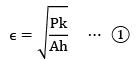and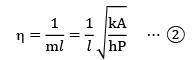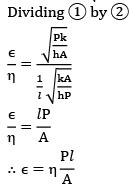QUESTION: 15

Consider a very hot small size copper ball dropped in cold water. The temperature of the ball drops down __________ with time.

Solution: The ball is very small in size and being made of copper it’s thermal conductivity is high. Therefore Biot’s number of the ball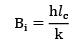is very less and it thus the ball can be treated as a lumped body. Based on lumped parameter analysis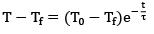i.e. temperature varies exponentially with time.

QUESTION: 16

The thermal conductivity of a solid depends upon the solid’s temperature as k = aT = + b where a and b are constants. The temperature in a planar layer of this solid as it conducts heat is given by

Solution: By heat conduction equation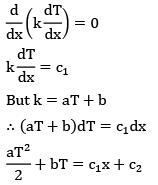QUESTION: 17

A plane wall of thickness 2L has a uniform volumetric heat source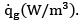It is exposed to local ambient temperature T at both the ends (x = ±L). The surface temperature Tg of the wall under steady-state condition (where h and k have their usual meanings) is given by

Solution: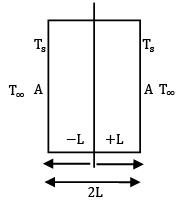In the given case the middle section will be the location of maximum temperature and heat generated in one half of the body will be dissipated from the corresponding surface.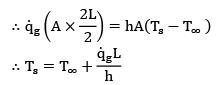QUESTION: 18

A very thin water jacketed copper rod ‘D’ m in diameter is used to carry current. The cooling water which flows continuously in the jacket, maintains the rod temperature at Ti°C during normal operation at ‘I’ amps. The electrical resistance of the rod is known to be R ?/m . If the coolant water ceased to be available and the heat removal rate diminished greatly, the rod would eventually melt. What is the time required for melting to start if the melting point of the rod material is Tmp? (cp is the specific heat and ρ is the density of the rod material.)

Solution: In the given situation, we can say that whatever heat is generated in the rod, it will be absorbed by the rod itself as there is no water to dissipate heat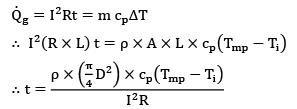QUESTION: 19

Two finned surfaces with long fins are identical, except that the convection heat transfer coefficient for the first finned surface is twice that of the second one. What statement below is accurate for the efficiency and effectiveness of the first finned surface relative to the second one?

Solution: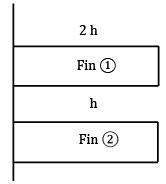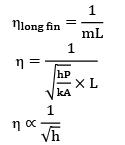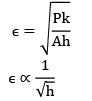∵ For fim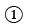the heat transfer coefficient is twice that of fin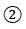so the effectiveness and efficiency both will be lower for fin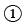as compare to finQUESTION: 20

The radial variation of temperature in an electrical wire carrying current is __________.

Solution: For cylindrical bodies with internal heat generation, heat conduction equation is given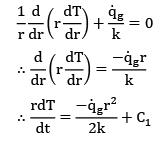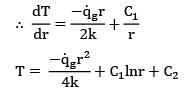As electrical wire is a solid body with defined temperature at r = 0, the equation is correct only if C1 = 0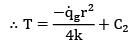i.e. T is a parabolic function of r